# Simulate a Chopper of Class A circuit in MATLAB Simulink

• Fatima Hasan

#### Fatima Hasan

Homework Statement
Attached below.
Relevant Equations
-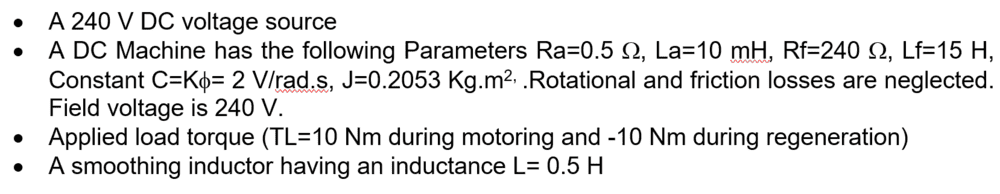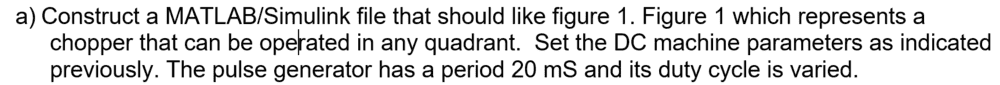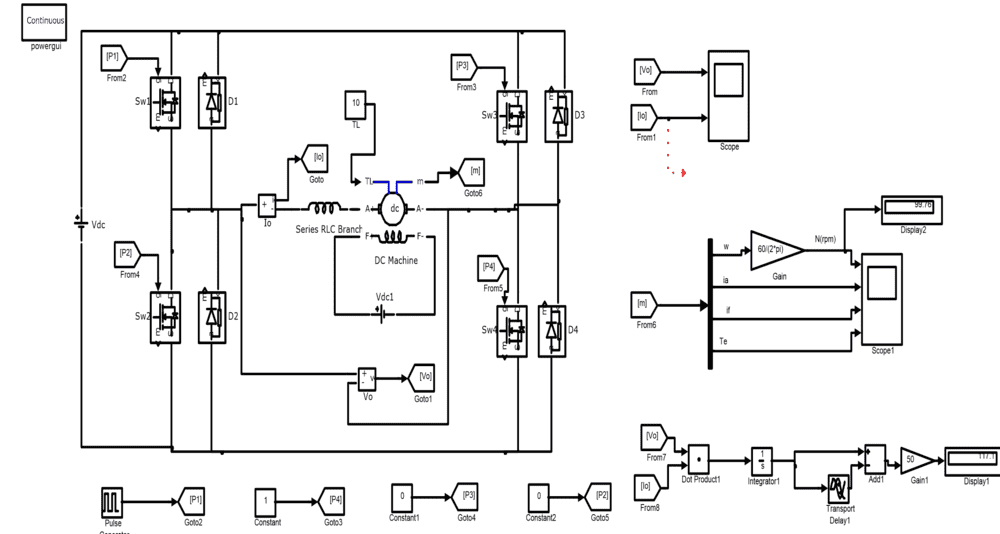I tried to simulate the circuit but I got unreliable results. Here's what I got when I set the duty cycle to be 20%:
Stop Time = 0.02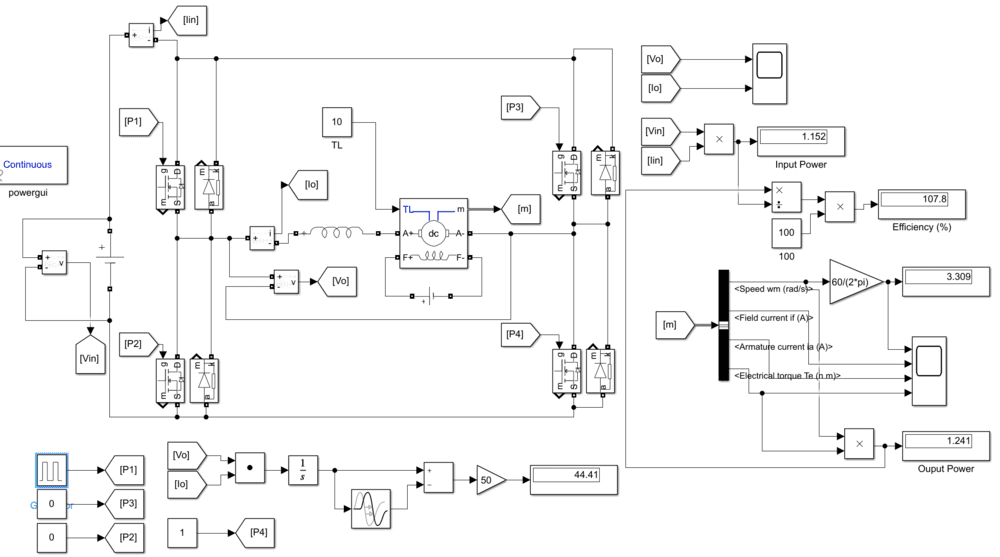The efficiency is more than100%.

Here's the parameters: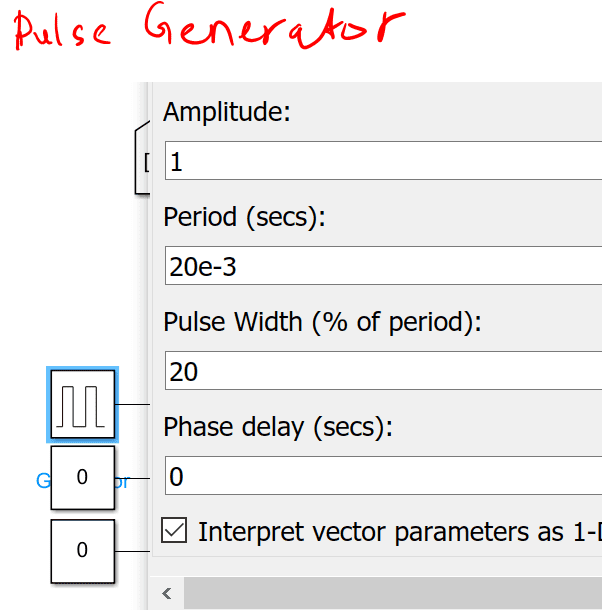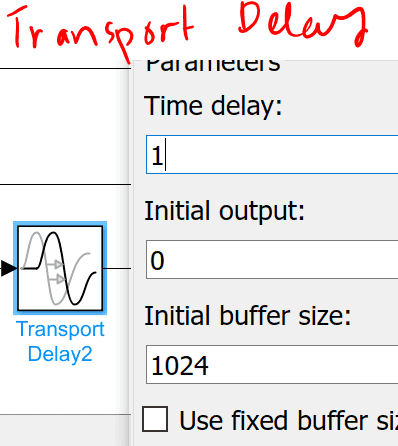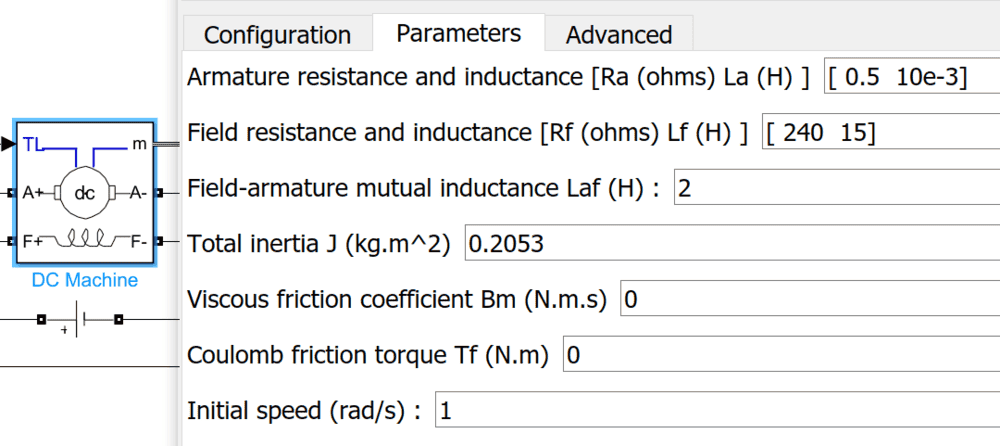Could someone please let me where is my mistake?

I'm definitely not an expert on this, but just looking at the numbers, these seem inconsistent.

What is the time constant of the Field winding?
How does that compare with the Stop Time?

I'm definitely not an expert on this, but just looking at the numbers, these seem inconsistent.

What is the time constant of the Field winding?
How does that compare with the Stop Time?
I modified the stop time and kept it equal to 10 sec. I got reasonable results. However, in the following part, I didn't.

To simulate a lift, let assume that the load torque is 20 Nm while going up and 10 Nm while going down as shown in figure 2(a). Also let the time of going up is 10 seconds and 10 seconds while going down. This can be done by gating the switches of figure 2 by the control blocks shown in figure 2(b).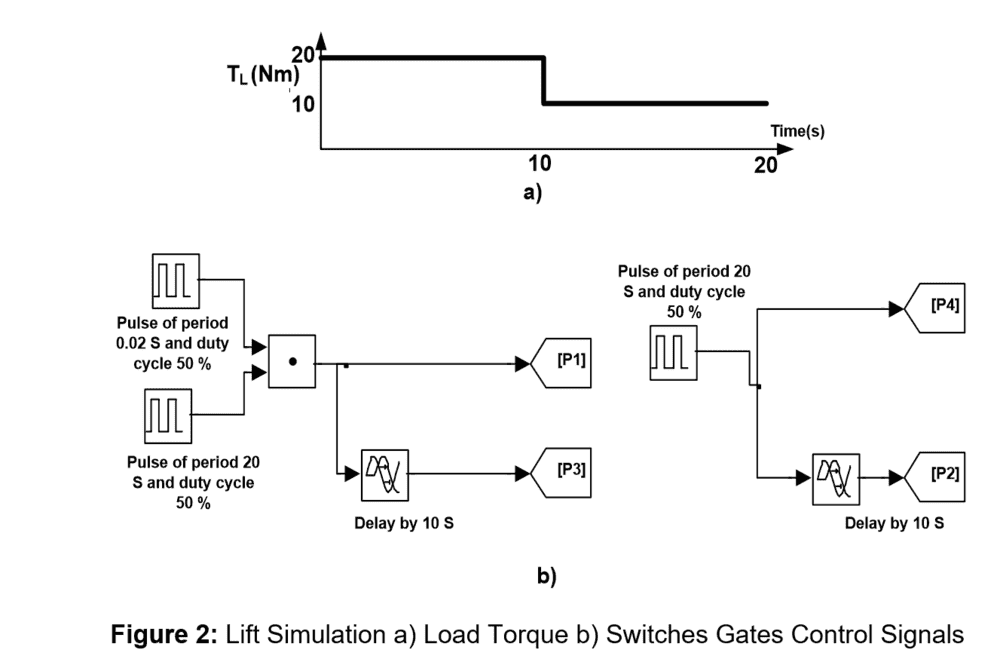Using figures 1 and 2, run the simulation for 20 s and record the machine speed, the power absorbed or generated by the machine, and the energy absorbed by the machine at times 9.999 seconds and 19.999 seconds respectively.

What are the differences of the speed and the output power in both cases (when t=9.999 and = 19.999 seconds) ?

#### Attachments

What are the differences of the speed and the output power in both cases (when t=9.999 and = 19.999 seconds) ?
I don't know, I haven't run the simulation and know nothing about either MATLAB or Simulink. I (think) I can understand the diagram fairly well and don't spot any major errors.

Something that stands out though is:
...let assume that the load torque is 20 Nm while going up and 10 Nm while going down...
But the problem statement says:
Applied load torque (TL=10nm during motoring and -10nm during regeneration)

...
Oh, Just spotted a questionable entry in the Parameters entries. Specifically the
Initial speed (rad/s) shows "1". Does this mean the motor is already running when the simulation starts?

Cheers,
Tom

Specifically the
Even when I changed it, the results didn't change.
Also, the energy in both cases are negative. Is that reasonable?

The only thing I see is that the circuit in post #1 is different than the attached circuit in post # 5. If you are using the the circuit in post #1, try the one in post #5, it may give more realistic results.

I just put in a request for help on this. It may take a couple days for a response, but SOMEONE almost always does!

Sorry I couldn't be of more help, it looked a little simpler when I first looked at the problem. :sad:

Hang in there, we'll get thru it!

Cheers,
Tom

Increase your stop time to say 50 secs. Initially, during transient time none of the values will be stable.

Secondly, don't you also need to include field winding voltage and current for input power calculation?

Thirdly, since you are input and/or output signals are chopped, you need to do an average the input and output power after the transient period and then calculate efficiency.

•Tom.G